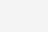# 基于单片机的led显示系统

2串LED背光的液晶屏需要超过6V的起辉电压，在3.3V-5V的单片机应用中需要升压才能点亮，若采用规范制式电路和器件来驱动会很嫌麻烦。但是你若不耻引用俺的招式，就会一下子简单易行、轻松愉快的搞定。void PWM_Init(void) //算法为f = fosc/12/256

{ PCA_USE_P24P25P26P27(); //切换输出引脚

CCON = 0; //初始化PCA控制寄存器

PCA_CLK_12T(); //fosc/12

PCA_PWM2 = 0x00; //PCA模块2工作于8位PWM,

CCAP2H = CCAP2L = 0xb0; //0x80时输出对称方波，增减可调空宽比。

CCAPM2 = 0x42; //PCA模块2为6位PWM模式

CR = 1; //PCA定时器开始工作

}

（若单片机没有推挽输出功能，则还需要加推挽输出管。）

timer0()interrupt 3 using 1

{ TR1 = 0;

TL1 = 0x00; //重设定时初值

TH1 = 0xC4; //重设定时初值

TR1 = 1; //定时器1开始计时

LCD_BG=~LCD_BG;

}

void main()

{ P4M0 =0x10;

P3M0=0x20;

AUXR &= 0xBF; //定时器时钟12T模式

TMOD &= 0x0F; //设置定时器模式

TMOD |= 0x10; //设置定时器模式

TL1 = 0x00; //设置定时初值

TH1 = 0xC4; //设置定时初值

EA=1; //CPU开中断*/

ET1=1; //T0开中断*/

TF1= 0; //清除TF1标志

TR1 = 1; //定时器1开始计时

。。。。。LED属于高速器件，这里用到5.5Khz，效果狠好、效率也高，还便于用较小电容驱动，蛮有利。### 相关推荐